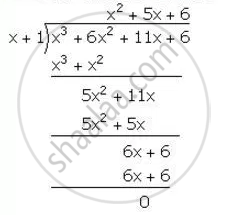Share

Factorise x3 + 6x2 + 11x + 6 completely using factor theorem. - ICSE Class 10 - Mathematics

ConceptFactorising a Polynomial Completely After Obtaining One Factor by Factor Theorem

Question

Factorise x3 + 6x2 + 11x + 6 completely using factor theorem.

Solution

"Let"  f(x)=x^3+6x^2+11x+6

for=x=-1

f(-1)=(-1)^3+6(-1)^2+11(-1)+6

=-1+6-11+6=12-12=0

Hence, (x+1) is a factory of f(x)∴ x^3+6x^2+11x+6=(x+1)(x^2+5x+6)

=(x+1)(x^2+2x+3x+6)

=(x+1)[x(x+2)+3(x+2)]

=(x+1)(x+2)(x+3)

Is there an error in this question or solution?

APPEARS IN

Solution Factorise x3 + 6x2 + 11x + 6 completely using factor theorem. Concept: Factorising a Polynomial Completely After Obtaining One Factor by Factor Theorem.
S